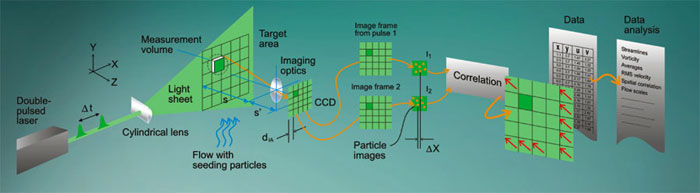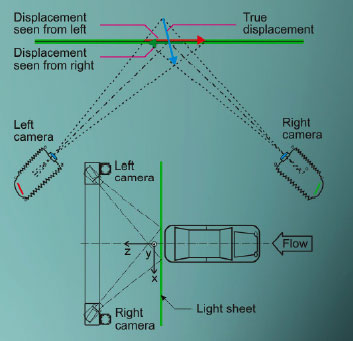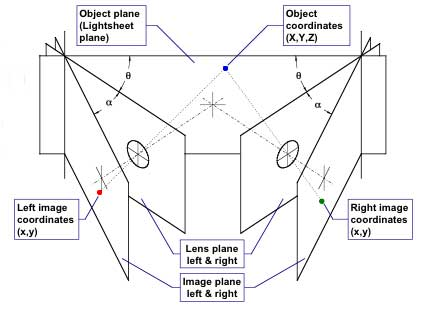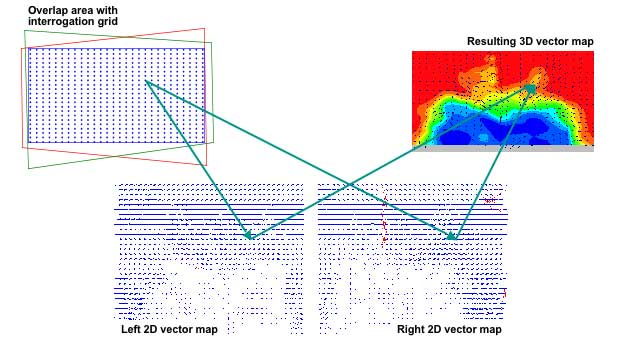Introduction

Particle Image Velocimetry (PIV) is a technique for gathering instantaneous velocity measurements in a cross-section (flat sheet) of flow. The use of two cameras for imaging allows the 3D flow information to be measured within the imaging plane. Each camera directly measures distances particles travel, and between these two images a third velocity direction can be computed to extrapolate 3D motion. With the right computer setup the measurement & display of information can be real time. The 'frame rate' for real-time setups is largely determined by the laser, which certain devices can measure flow rates at more than 20,000 frames per second. At these speeds the imaging volume / area is decreased - so an experimenter trades off between resolution, area, and cost.The technique divides the image data into discrete areas, then records the change in pixel values within that area dure the experiment. Higher frame rates allow sub-pixel interpolation as particles move 'between pixels' on the camera, generating very precise information about velocity vectors (direction). For each area being computed, about 10-25 images are used. Attention must be given to the estimated velocities in the system, as particles traveling fast enough to cover more area than the discrete areas being computed will not be properly tracked - and in extreme circumstances can through off measurements in a significant manner. Choosing larger discrete areas for measurement increases computation requirements, and can disallow real-time measurement.Measurement must account for the image plane of the CCDs (which converts light into electrical signals), the lens plane of the cameras and the object plane of the laser light sheet. The overlapping areas of each image are then masked in and changes in the image between frames allows particle velocities to be known.